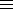### Calculating a p-adic square root, p an odd prime

This returns the first n p-adic digits of the two p-adic sqroots x of a quadratic residue a (mod p).
Here p is an odd prime and x ≡ b (mod p), where b2 ≡ a (mod p), 0 < b < p.
This is a BCMATH translation of a BC program.
A sequence of positive integers an < pn+1, n ≥ 0 is constructed, which converges p-adically to a p-adic square root of a:
a0 = b and an = an-1 + bnpn, n ≥ 1.
an-12 ≡ a (mod pn), k = (an-12 - a)/pn,
k + 2an-1bn ≡ 0 (mod p), 0 ≤ bn < p.
Then the two p-adic square roots of a are a0 + b1p + ···

Also see lecture notes and solutions.

Enter a (non-zero):
Enter n (> 0):
Enter p (an odd prime), where a(p-1)/21(mod p):